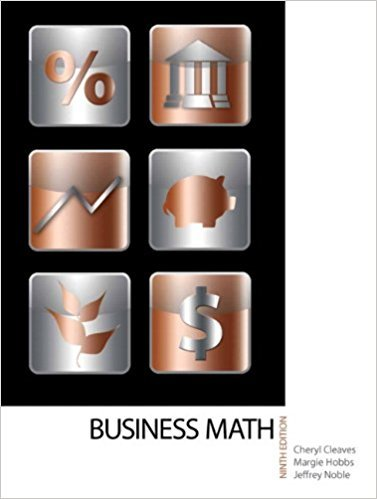×
×

# Solutions for Chapter 5-3: FORMULAS## Full solutions for Business Math, | 9th Edition

ISBN: 9780135108178Solutions for Chapter 5-3: FORMULAS

Solutions for Chapter 5-3
4 5 0 432 Reviews
24
2
##### ISBN: 9780135108178

Since 12 problems in chapter 5-3: FORMULAS have been answered, more than 19264 students have viewed full step-by-step solutions from this chapter. Chapter 5-3: FORMULAS includes 12 full step-by-step solutions. This expansive textbook survival guide covers the following chapters and their solutions. Business Math, was written by and is associated to the ISBN: 9780135108178. This textbook survival guide was created for the textbook: Business Math, , edition: 9.

Key Math Terms and definitions covered in this textbook
• Associative Law (AB)C = A(BC).

Parentheses can be removed to leave ABC.

• Augmented matrix [A b].

Ax = b is solvable when b is in the column space of A; then [A b] has the same rank as A. Elimination on [A b] keeps equations correct.

• Cholesky factorization

A = CTC = (L.J]))(L.J]))T for positive definite A.

• Complex conjugate

z = a - ib for any complex number z = a + ib. Then zz = Iz12.

• Determinant IAI = det(A).

Defined by det I = 1, sign reversal for row exchange, and linearity in each row. Then IAI = 0 when A is singular. Also IABI = IAIIBI and

• Dimension of vector space

dim(V) = number of vectors in any basis for V.

• Eigenvalue A and eigenvector x.

Ax = AX with x#-O so det(A - AI) = o.

• Fundamental Theorem.

The nullspace N (A) and row space C (AT) are orthogonal complements in Rn(perpendicular from Ax = 0 with dimensions rand n - r). Applied to AT, the column space C(A) is the orthogonal complement of N(AT) in Rm.

• Inverse matrix A-I.

Square matrix with A-I A = I and AA-l = I. No inverse if det A = 0 and rank(A) < n and Ax = 0 for a nonzero vector x. The inverses of AB and AT are B-1 A-I and (A-I)T. Cofactor formula (A-l)ij = Cji! detA.

• Linear combination cv + d w or L C jV j.

• Norm

IIA II. The ".e 2 norm" of A is the maximum ratio II Ax II/l1x II = O"max· Then II Ax II < IIAllllxll and IIABII < IIAIIIIBII and IIA + BII < IIAII + IIBII. Frobenius norm IIAII} = L La~. The.e 1 and.e oo norms are largest column and row sums of laij I.

• Nullspace matrix N.

The columns of N are the n - r special solutions to As = O.

• Permutation matrix P.

There are n! orders of 1, ... , n. The n! P 's have the rows of I in those orders. P A puts the rows of A in the same order. P is even or odd (det P = 1 or -1) based on the number of row exchanges to reach I.

• Saddle point of I(x}, ... ,xn ).

A point where the first derivatives of I are zero and the second derivative matrix (a2 II aXi ax j = Hessian matrix) is indefinite.

• Semidefinite matrix A.

(Positive) semidefinite: all x T Ax > 0, all A > 0; A = any RT R.

• Similar matrices A and B.

Every B = M-I AM has the same eigenvalues as A.

• Spanning set.

Combinations of VI, ... ,Vm fill the space. The columns of A span C (A)!

• Spectral Theorem A = QAQT.

Real symmetric A has real A'S and orthonormal q's.

• Stiffness matrix

If x gives the movements of the nodes, K x gives the internal forces. K = ATe A where C has spring constants from Hooke's Law and Ax = stretching.

• Unitary matrix UH = U T = U-I.

Orthonormal columns (complex analog of Q).

×